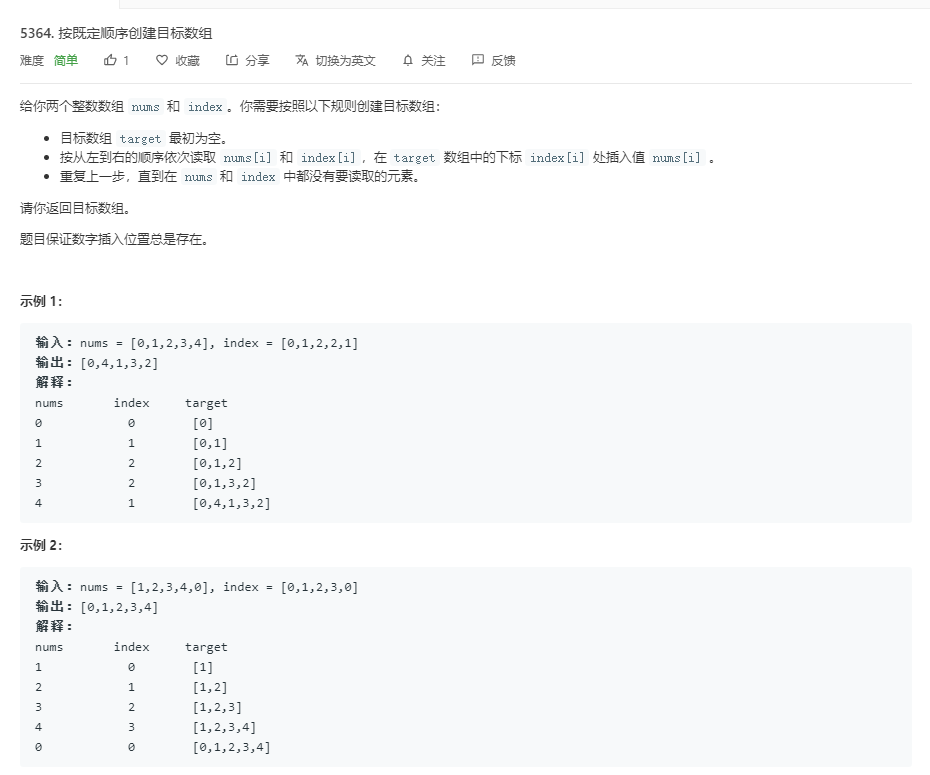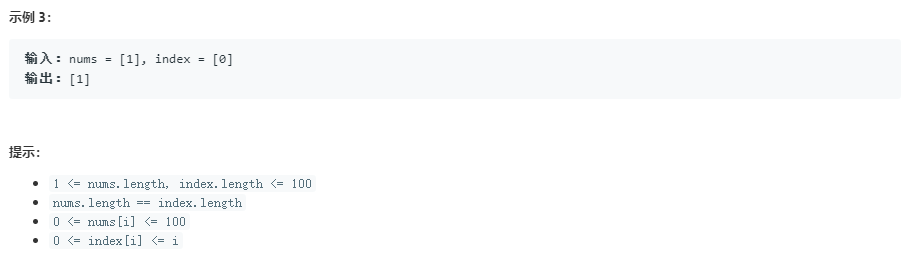## 2. 题目说明## 3. 题目解析

### 方法一：暴力+常规解法

``````// 执行用时 :0 ms, 在所有 C++ 提交中击败了100.00%的用户
// 内存消耗 :9.9 MB, 在所有 C++ 提交中击败了100.00%的用户

class Solution {
public:
vector<int> createTargetArray(vector<int>& nums, vector<int>& index) {
vector<int> vt;
for (int i = 0; i < nums.size(); ++i) {
int a = index[i];
int x = nums[i];
vt.insert(vt.begin() + a, x);
}
return vt;
}
};
``````